# How to create interactive data visualization using plotly

Visualization is the graphical representation of your data and it let you paint your data into a canvas in a way you want to see it. There are lot of amazing libraries and tools available in the market to plot your data without much of effort

However being a Data Scientist, I mostly work on Python and always looking around the amazing open source tools developed by those amazing peoples and leveraging their power in my work

In my previous post about Data Visualization, I explained how flexible is the Pandas plot function which is a wrapper of matplotlib and can be used with much ease to give a graphical shape to your data in no time.

Recently, I used plotly for some visualization work and found it to be a great tool for visualizing your data and gives a quick turnaround

## What is plotly?

Its a graphing library that lets you create an interactive graphs on your browser using python and You can also view it on a jupyter notebook or a HTML file

## Installation

As of writing this post 4.4.1 is the latest stable version of plotly

Install it using pip command:

``````pip install plotly==4.4.1
``````

## Getting Started

I am using the World Happiness index data of 2019 to plot different graphs type and to explore plotly functions.

## Create Dataframe

We will first create a dataframe of downloaded data because we will be using this dataframe for plotting in the following sections

``````import pandas as pd
``````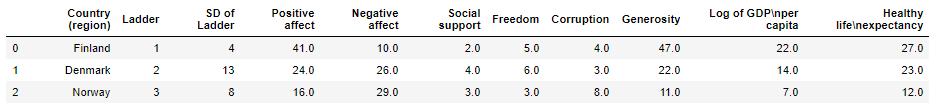Rename Columns:

The original column names are long therefore we will rename those columns to something meaningful

``````df.rename(columns={"Country (region)": "Country", "Log of GDPnper capita": "Log_GDP_per_capita",
"Healthy lifenexpectancy":"Health_life_expect"},inplace=True)
``````

## Plotly Bar Chart

We will plot the columns in group for the top 5 happiest country and will display them side-by-side

We are creating an array of top 5 happiest country and then adding plotly graph object Bar for each of the columns in a data array

The argument x is the array of country and argument y is the pandas series object of each of the column

You can also create this data array using a for loop

Finally we will update the layout of the figure and pass the barmode parameter as group to create a grouped bar graph

``````import plotly as pt
import plotly.graph_objs as go
country =df[:5]['Country']

fig = go.Figure(data=[
go.Bar(name='Corruption', x=country, y=df[:5]['Corruption']),
go.Bar(name='Freedom', x=country, y=df[:5]['Freedom']),
go.Bar(name='Generosity', x=country, y=df[:5]['Generosity']),
go.Bar(name='Social support', x=country, y=df[:5]['Social support'])
])

# Change the bar mode
fig.layout.update(barmode='group')
fig
``````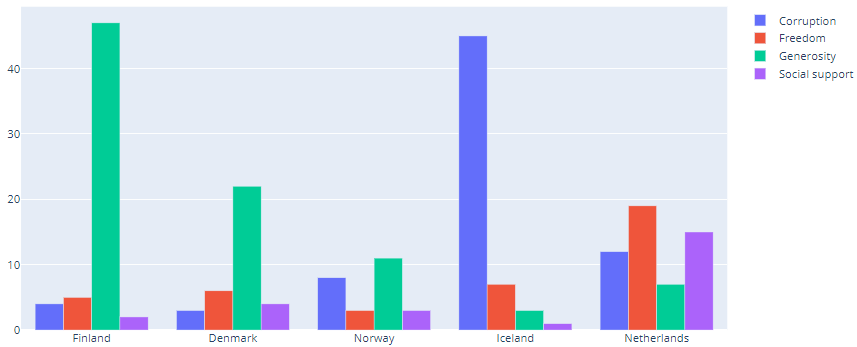## Line chart

We are first selecting the first five rows from the dataframe to get the top five happiest country and then plot Country as x-axis and other five columns – Corruption, Freedom, Generosity, Social support as y-axis

Finally changing the plotly graph object to Line.

The four columns are also shown in the legends box

``````import plotly as pt
import plotly.graph_objs as go
country =df[:5]['Country']

fig = go.Figure(data=[
go.Line(name='Corruption', x=country, y=df[:5]['Corruption']),
go.Line(name='Freedom', x=country, y=df[:5]['Freedom']),
go.Line(name='Generosity', x=country, y=df[:5]['Generosity']),
go.Line(name='Social support', x=country, y=df[:5]['Social support'])
])

# Change the bar mode
fig.layout.update(barmode='group')
fig.show()
``````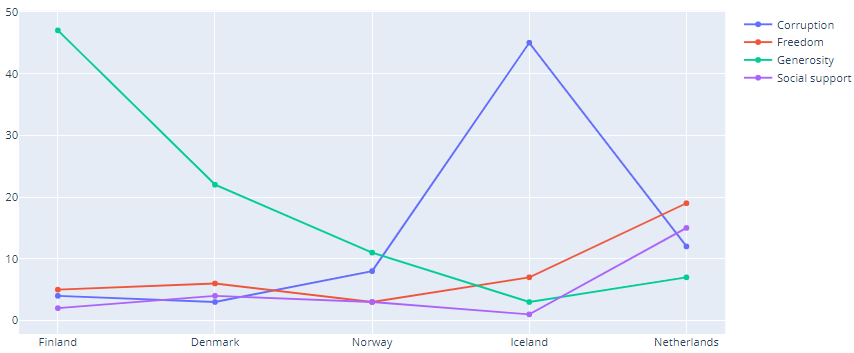We will plot the box graph now and this time we will update the figure object using the add_trace() method

New traces can be added to a graph object figure using the `add_trace` method.

This method accepts a graph object trace (an instance of `go.Scatter``go.Bar`, etc.) and adds it to the figure.

``````import plotly.graph_objects as go
import numpy as np

fig = go.Figure()
for items in df.columns[1:]:
fig.show()
``````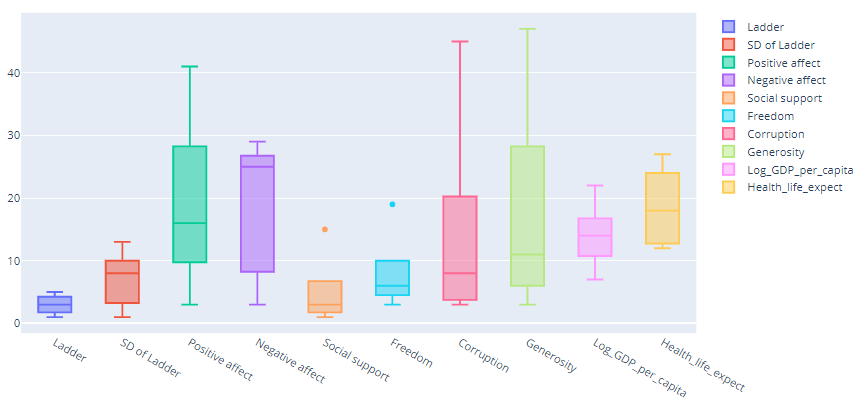## Scatter Plot

We will create the scatter plot using Plotly Express which is easy-to-use, high-level interface to Plotly

With `px.scatter`, each data point is represented as a marker point, which location is given by the `x` and `y` columns

The `color` data is added to hover information. You can add other columns to hover data with the `hover_data` argument of `px.scatter`

``````import plotly.express as px
fig = px.scatter(df,x= "Corruption",y= "Generosity",color='Corruption')
fig.show()
``````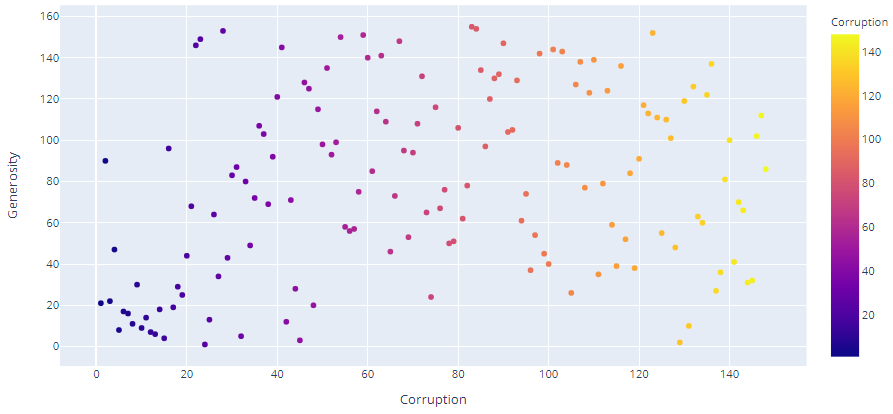## Plotly express Scatter Matrix

As per the definition in official plotly documentation:

A scatterplot matrix is a matrix associated to n numerical arrays (data variables), X1,X2,…,XnX1,X2,…,Xn , of the same length. The cell (i,j) of such a matrix displays the scatter plot of the variable Xi versus Xj.

We are using the plotly express scatter_matrix function to plot the first four columns of the dataframe excluding Country column

We can specify the columns to be displayed in the dimensions parameter and represent color using any of the column

``````fig = px.scatter_matrix(df[:10], dimensions=df.columns[1:5], color="Country")
fig.show()
``````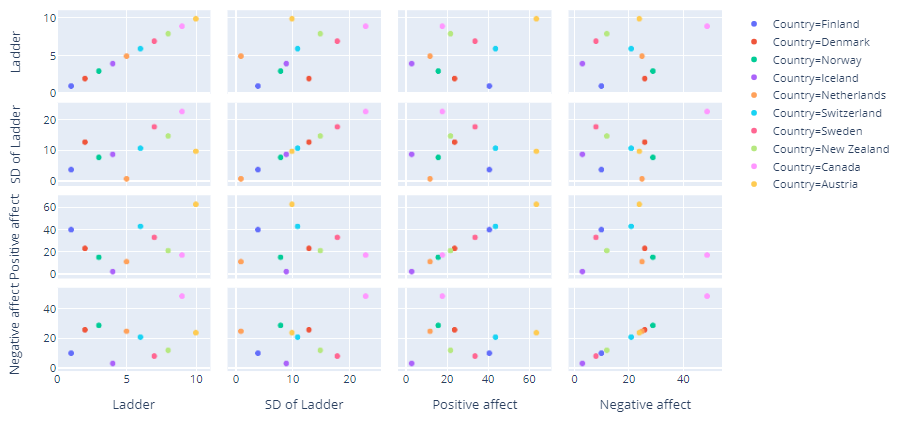## Plotly X and Y axis Range

In this below line chart we will set x limit from 0 to 20 and y limit from 0 to 100

we are slicing the original dataframe(df[:20]) to get first 20 happiest countries and

then use `**Line**` function with X-axis set as numpy ndarray with range of 100 and Y-axis set as Array of Freedom column for first top 20 highest country

We are updating the figure x axis and y axis by setting the start value of the axes and the interval

So for x-axis the start value is 0 and interval is 1 and for y-axis the start value is 0 and interval is 20 and that is evident from the plot below

``````fig = go.Figure(data=[
go.Line(x=np.arange(101),y=df[:20]['Freedom'])])

# Change the bar mode
fig.update_yaxes(tick0=0, dtick=20)
fig.update_xaxes(tick0=0, dtick=1)
fig.show()
``````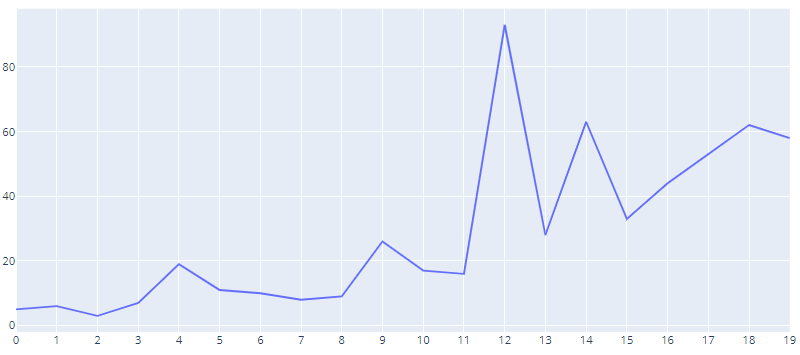## Plotly tickvals

You can also set your own tick value array and pass it to tickvals parameter

Because we are passing [0,5,10,15,20] array to the tickvals argument in the update_xaxes() function the plot shows the same values for the x-axis

``````fig = go.Figure(data=[
go.Line(x=np.arange(101),y=df[:20]['Freedom'])])

# Change the bar mode
fig.update_yaxes(tick0=0, dtick=20)
fig.update_xaxes(tickvals=[0,5,10,15,20])
fig.show()
``````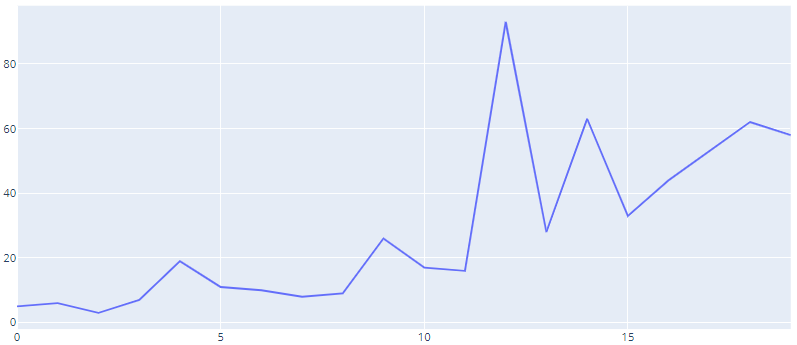## Plotly axis tick labels

We can also customize the tick marks in the plot by setting the tick width, color and length arguments in update functions

``````fig = go.Figure(data=[
go.Line(x=np.arange(101),y=df[:20]['Freedom'])])

# Change the bar mode
fig.update_yaxes(tick0=0, dtick=20)
fig.update_xaxes(tickvals=[0,5,10,15,20],ticks="inside", tickwidth=1, tickcolor='black', ticklen=20)
fig.show()
``````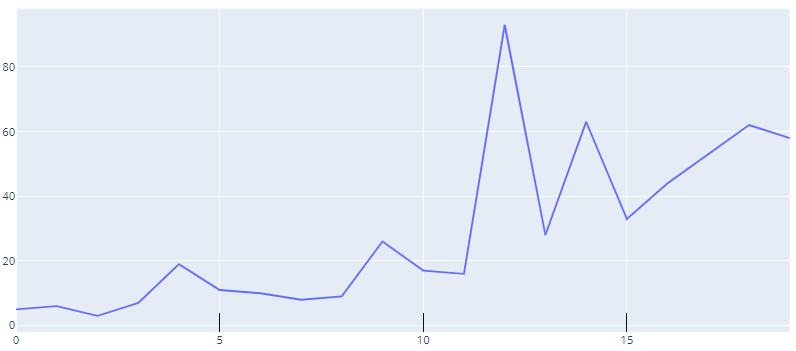We can also style the line plots like color and dash of the traces, adds trace names, modifies line width, and adds plot and axes titles

So here we are just updating the line as dashdot, Beside you can also set it as dash or dot and set the width and color of the line by passing the dict in line argument

``````fig = go.Figure()

fig.show()
``````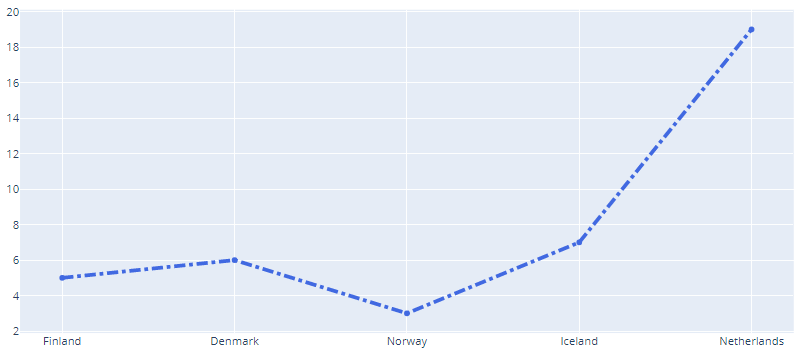## Update Layout

We can update the layout by giving a title to the plot and naming it’s x-axis and y-axis because that gives more info about your chart and it’s axes

``````fig = go.Figure()
line=dict(color='royalblue', width=4,dash='dashdot'))) ## dash options include 'dash', 'dot', and 'dashdot'

fig.update_layout(title='Country-wise Freedom',
xaxis_title='Country',
yaxis_title='Freedom')
fig.show()
``````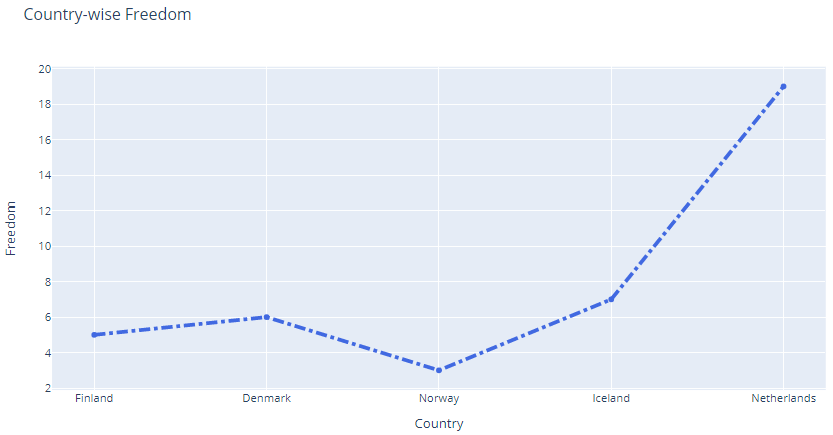## Line Shape

Because in the above line chart you have not set the line_shape argument so by default it was linear

However if you want to select some other shape for the lines then select from the following options: linear, spline, vhv, hvh,vh, hv

We have selected a line_shape hvh here

``````fig = go.Figure()
line=dict(color='royalblue', width=4,dash='dashdot'),line_shape='hvh')) ## dash options include 'dash', 'dot', and 'dashdot'

fig.show()
``````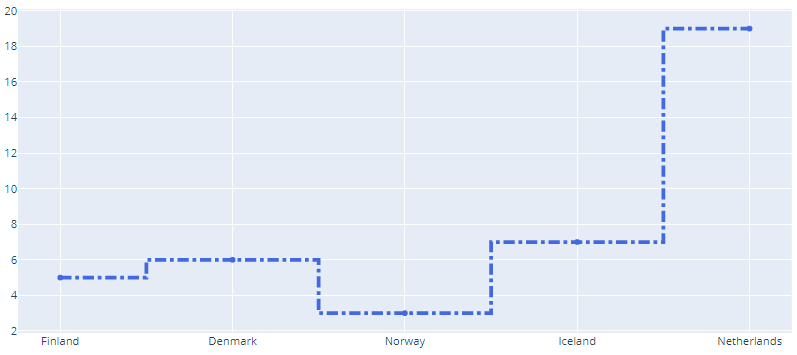## Stacked Bar

We will create the stacked bar containing all the columns of top 5 happiest country

Just make sure to change the barmode argument to stack

``````country=df[:5]['Country']
data = []

for items in df.columns[1:]:
data.append(go.Bar(name=items, x=country, y=df[:5][items]))

fig = go.Figure(data=data)
# Change the bar mode
fig.update_layout(barmode='stack')
fig.show()
``````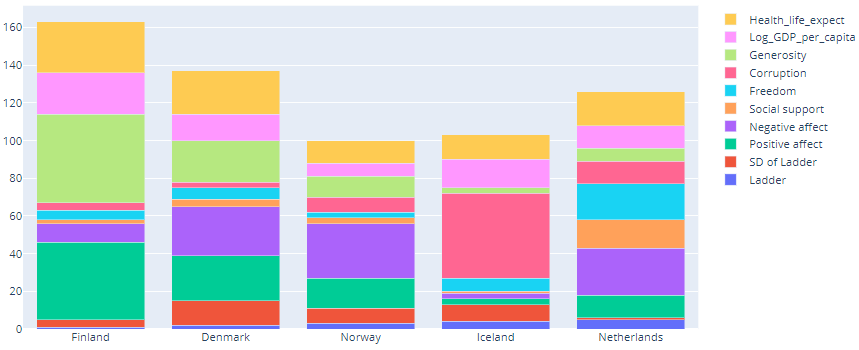## Grid Lines

So you want to see the axis grid lines because that gives a more feel about the scales in plot

So, update the axes and set the showgrid argument as True and beside that set other arguments like gridwidth and gridcolor

``````fig = go.Figure()
line=dict(color='royalblue', width=4)))

fig.update_xaxes(showgrid=True, gridwidth=1, gridcolor='Green')
fig.update_yaxes(showgrid=True, gridwidth=1, gridcolor='Green')
fig.show()
``````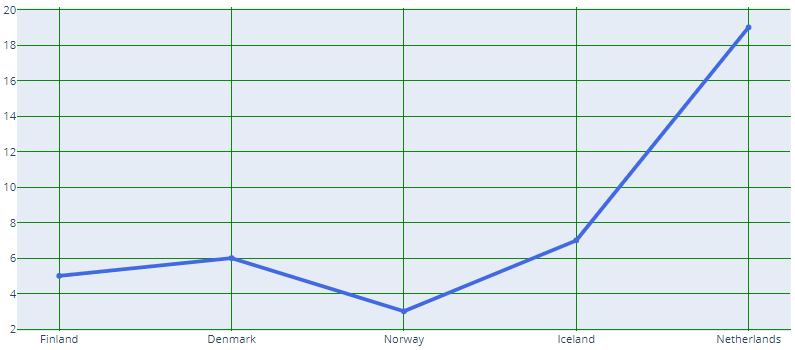## Plotly Sub Plots

With `**subplot**` you can arrange plots in a regular grid.

You need to specify the position by setting the row and column number for each of the plot

In the below subplot we are creating the histogram of four columns

``````from plotly.subplots import make_subplots
import plotly.graph_objects as go

fig = make_subplots(
rows=2, cols=2,
subplot_titles=("Corruption Hist", "Freedom Hist", "Social support Hist", "Generosity Hist"))

row=1, col=1)

row=1, col=2)

row=2, col=1)

row=2, col=2)

fig.update_layout(height=500, width=700,
title_text="Multiple Subplots with Titles")

fig.show()
``````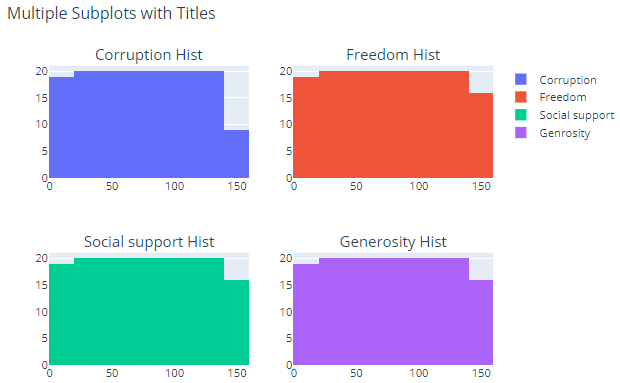## Conclusion

In this post we have seen how plotly can be used easily to visualize your data and create an eye catching plots in no time.

Most Importantly, We have learnt to create different types of plots - Bar, Scatter, Stacked bar and Line. Additionally we’ve seen how to customize the layout and make your graphs beautiful by changing the color and scale.

With plotly you can also set the line and it’s properties and create subplots with different columns of your data

Tags:

Categories:

Updated: